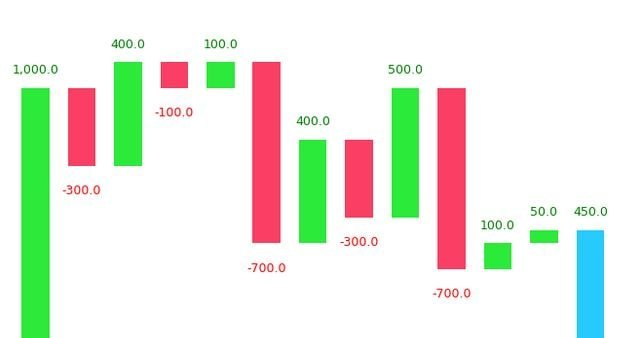Drop a Query

# plots## Matplotlib Subplots – How to create multiple plots in same figure in Python?

Subplots mean groups of axes that can exist in a single matplotlib figure. subplots() function in the matplotlib library, helps in creating multiple layouts of subplots. It provides control over all the individual plots that are created. Matplotlib Subplots in Python CONTENTS Basic Overview axes() function add_axis() function Creating multiple grids in the same graph …## Waterfall Plot in Python

Waterfall chart is a 2D plot that is used to understand the effects of adding positive or negative values over time or over multiple steps or a variable. Waterfall chart is frequently used in financial analysis to understand the gain and loss contributions of multiple factors over a particular asset. Contents Introduction Simple Waterfall Plot …## Matplotlib Line Plot – How to create a line plot to visualize the trend?

Line plot is a type of chart that displays information as a series of data points connected by straight line segments. A line plot is often the first plot of choice to visualize any time series data. Contents What is line plot? Simple Line Plot Multiple Line Plot in the same graph Creating a secondary …## Python Scatter Plot – How to visualize relationship between two numeric features

Scatter plot is a graph in which the values of two variables are plotted along two axes. It is a most basic type of plot that helps you visualize the relationship between two variables. Concept What is a Scatter plot? Basic Scatter plot in python Correlation with Scatter plot Changing the color of groups of …Course Preview

## Machine Learning A-Z™: Hands-On Python & R In Data Science

### Free Sample Videos:#### Machine Learning A-Z™: Hands-On Python & R In Data Science#### Machine Learning A-Z™: Hands-On Python & R In Data Science#### Machine Learning A-Z™: Hands-On Python & R In Data Science#### Machine Learning A-Z™: Hands-On Python & R In Data Science